# 1 Getting started

The SEtools package is a set of convenience functions for the Bioconductor class SummarizedExperiment. It facilitates merging, melting, and plotting `SummarizedExperiment` objects.

## 1.1 Package installation

``````if (!requireNamespace("BiocManager", quietly = TRUE))
install.packages("BiocManager")
BiocManager::install("SEtools")``````

Or, to install the latest development version:

``BiocManager::install("plger/SEtools")``

## 1.2 Example data

To showcase the main functions, we will use an example object which contains (a subset of) whole-hippocampus RNAseq of mice after different stressors:

``````suppressPackageStartupMessages({
library(SummarizedExperiment)
library(SEtools)
})``````
``````## Warning: replacing previous import 'ComplexHeatmap::pheatmap' by
``````data("SE", package="SEtools")
SE``````
``````## class: SummarizedExperiment
## dim: 100 20
## assays(2): counts logcpm
## rownames(100): Egr1 Nr4a1 ... CH36-200G6.4 Bhlhe22
## rowData names(2): meanCPM meanTPM
## colnames(20): HC.Homecage.1 HC.Homecage.2 ... HC.Swim.4 HC.Swim.5
## colData names(2): Region Condition``````

This is taken from Floriou-Servou et al., Biol Psychiatry 2018.

## 1.3 Heatmaps

There are two main wrappers for plotting heatmaps from `SummarizedExperiment` objects:

• the `sehm` function uses the pheatmap engine
• the `sechm` function uses the ComplexHeatmap engine

Both functions were made to function very similarly, but the `sechm` function is especially useful to combine heatmaps (for instance, from different `SummarizedExperiment` objects). We’ll showcase `sehm` (the main functionalities being replicable with `sechm`), and will then provide examples of multiple heatmaps.

### 1.3.1 sehm

The `sehm` function simplifies the generation of heatmaps from `SummarizedExperiment`. It uses pheatmap, so any argument supported by it can in principle be passed:

``````g <- c("Egr1", "Nr4a1", "Fos", "Egr2", "Sgk1", "Arc", "Dusp1", "Fosb", "Sik1")
sehm(SE, genes=g)``````
``## Using assay logcpm``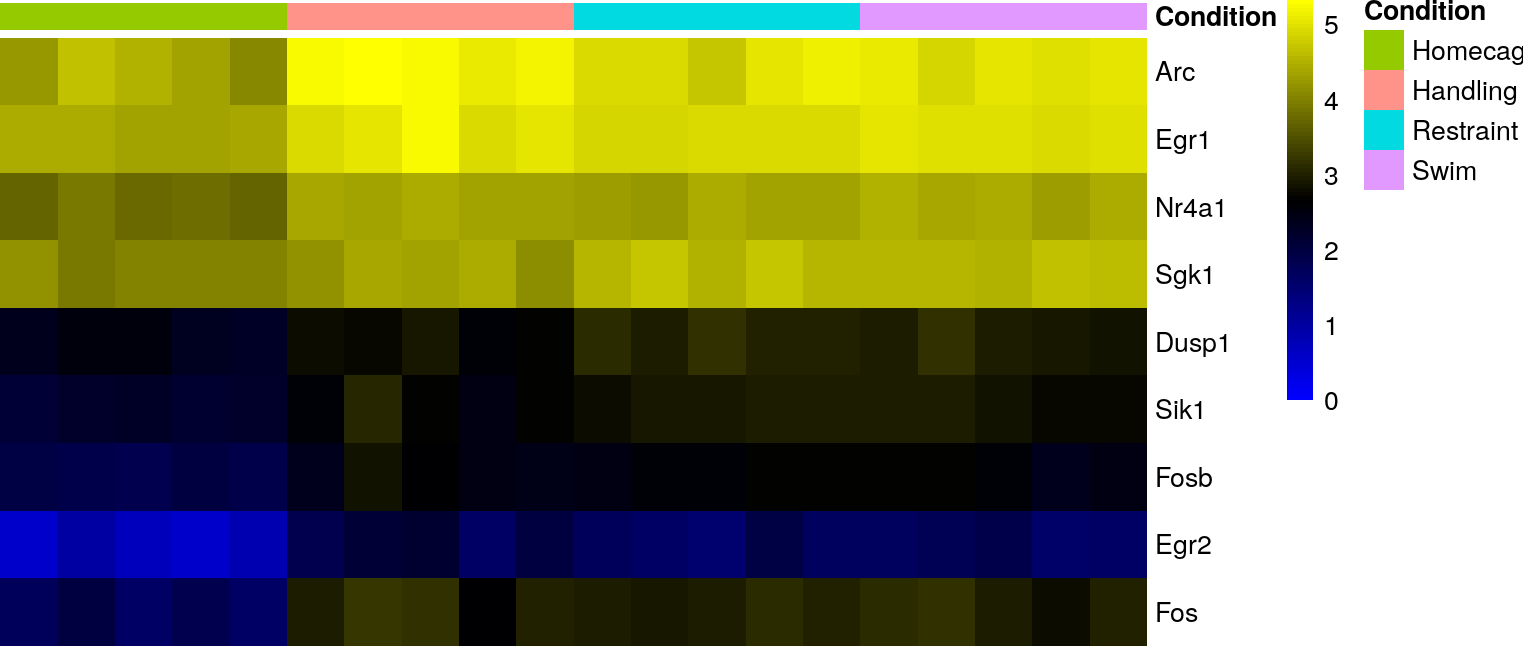``sehm(SE, assayName="logcpm", genes=g, do.scale=TRUE)``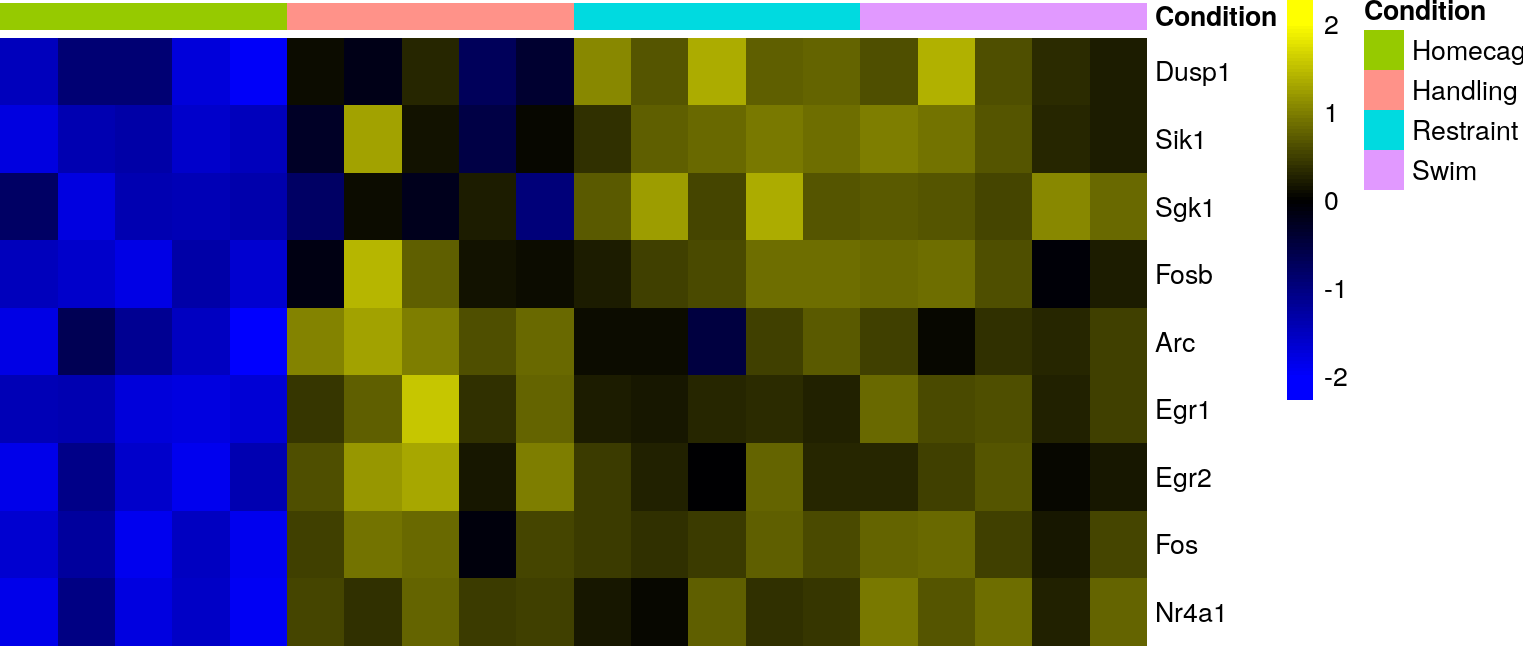When scaling data, the function will automatically center the colour scale around zero, and handle the extreme values (0.5% percentile on each side) in a non-linear fashion to retain a useful visualization. This behavior can be manually controlled via the `breaks` parameter (either setting it to FALSE, to a percentile until which the scale should be linear, of manually inputting breaks).

Annotation from the object’s `rowData` and `colData` can be plotted simply by specifying the column name (some will be shown by default if found):

``sehm(SE, assayName="logcpm", genes=g, do.scale=TRUE, anno_rows="meanTPM")``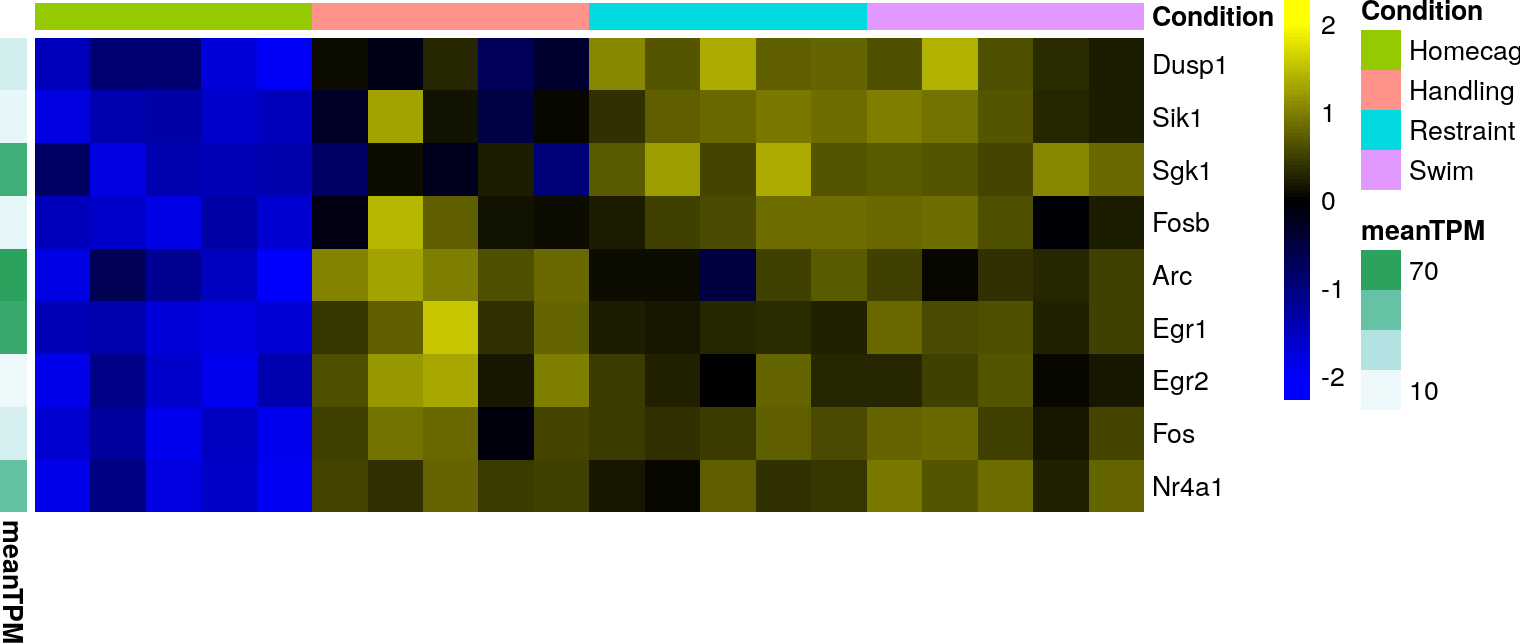These can also be used to create gaps:

``sehm(SE, genes=g, do.scale=TRUE, anno_rows="meanTPM", gaps_at="Condition")``
``## Using assay logcpm``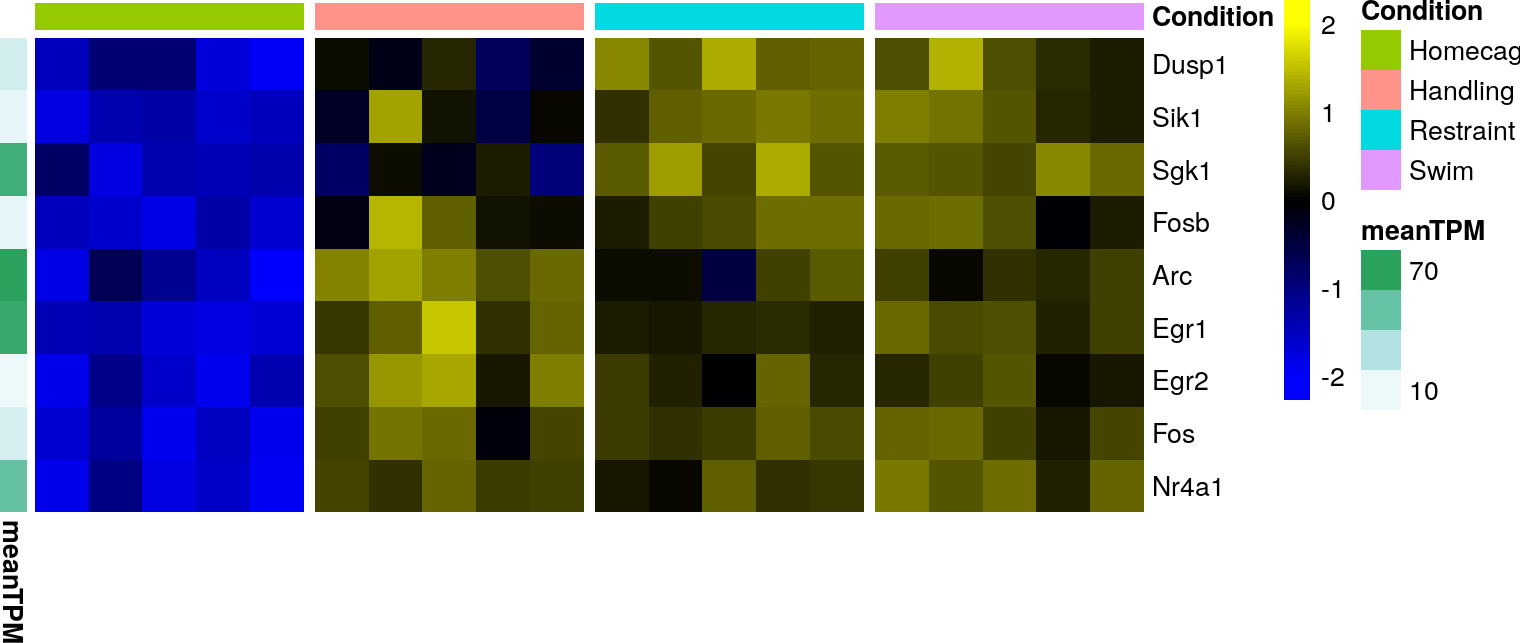The specific assay to use for plotting can be specified with the `assayName` argument.

#### 1.3.1.1 Row/column ordering

By default, rows are sorted not with hierarchical clustering, but from the angle on a MDS plot, which tends to give nicer results than bottom-up hierarchical clustering. This can be disabled using `sortRowsOn=NULL` or `cluster_rows=TRUE` (to avoid any row reordering and use the order given, use `sortRowsOn=NULL, cluster_rows=FALSE`). Column clustering is disabled by default, but this can be changed with `cluster_cols=TRUE`.

It is common to cluster features into groups, and such a clustering can be used simultaneously with row sorting using the `toporder` argument. For instance:

``````lfcs <- assays(SE)\$logcpm-rowMeans(assays(SE)\$logcpm[,which(SE\$Condition=="Homecage")])
rowData(SE)\$cluster <- as.character(kmeans(lfcs,4)\$cluster)
sehm(SE, genes=g, do.scale=TRUE, anno_rows="cluster", toporder="cluster", gaps_at="Condition")``````
``## Using assay logcpm``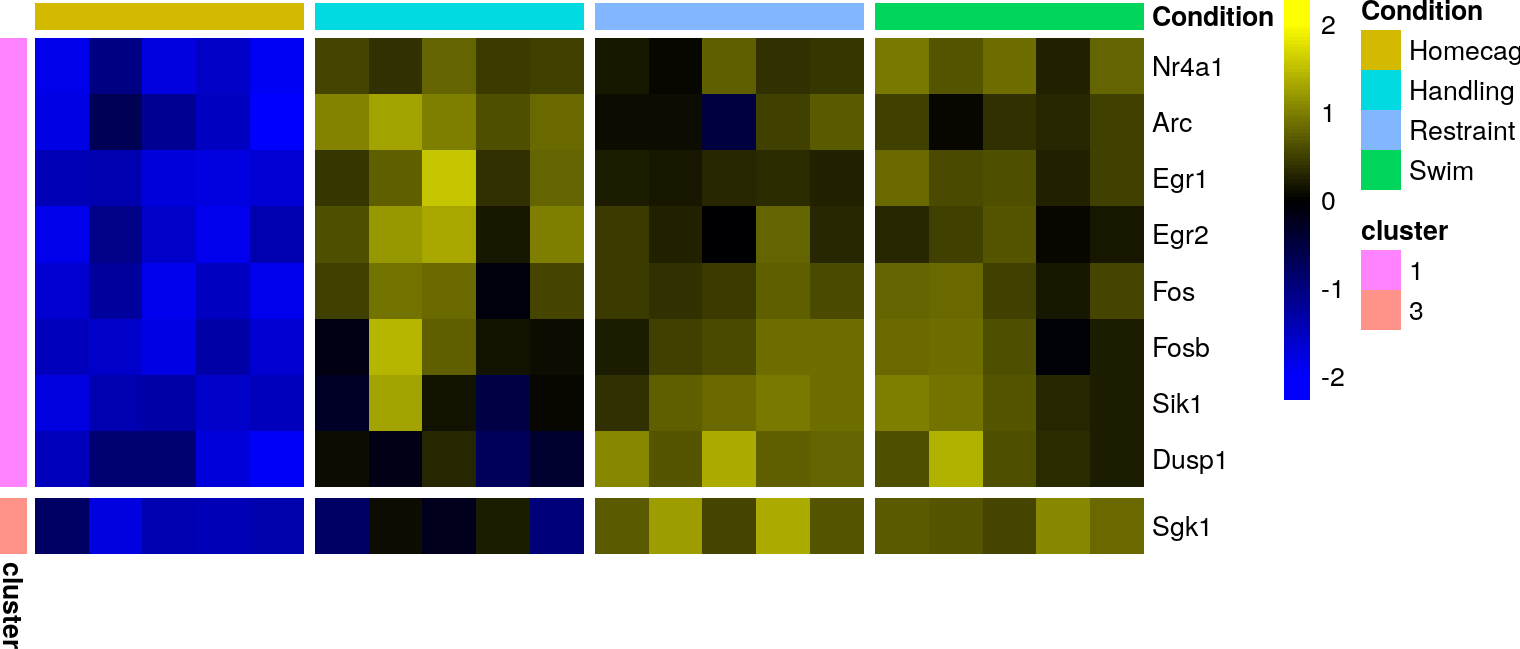#### 1.3.1.2 Default arguments

For some arguments (for instance colors), if they are not specified in the function call, `SEtools` will try to see whether the object itself contains it, or whether the corresponding global options have been set, before using default colors. This means that if, in the context of a given project, the same colors are repeatedly being used, they can be specified a single time, and all subsequent plots will be affected.

Storing colors in the object:

``````metadata(SE)\$hmcols <- c("purple","white","gold")
ancols <- list( Condition=c( Homecage="#DB918B",
Handling="#B86FD3",
Restraint="#A9CED5",
Swim="#B5DF7C" ) )
sehm(SE, g, do.scale = TRUE)``````
``## Using assay logcpm``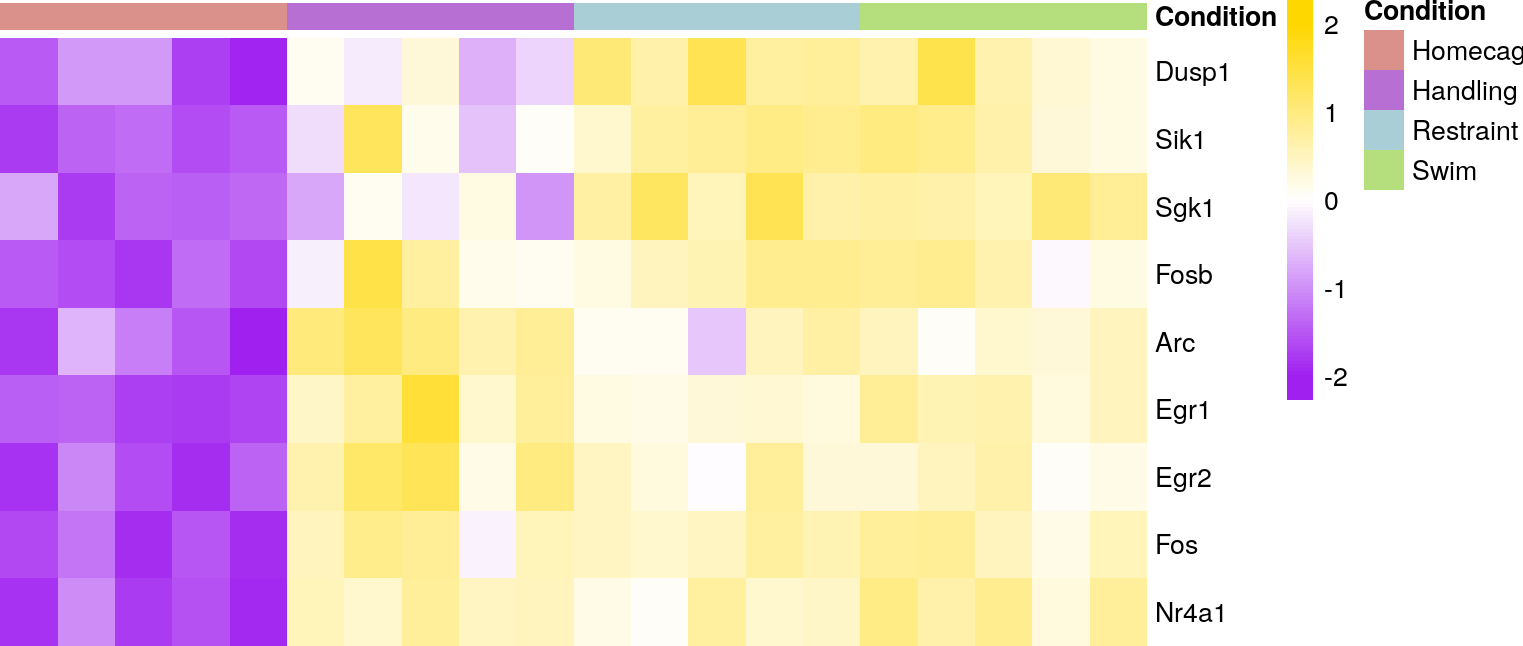Using the global options:

``````options("SEtools_def_hmcols"=c("white","grey","black"))
options("SEtools_def_anno_colors"=ancols)
sehm(SE, g, do.scale = TRUE)``````
``## Using assay logcpm``At the moment, the following arguments can be set as global options: `assayName`, `hmcols`, `anno_columns`, `anno_rows`, `anno_colors`, `gaps_at`, `breaks`. Options must be set with the prefix `SEtools_def_`, followed by the name of the argument.

To remove the predefined colors:

``````resetAllSEtoolsOptions()

In order of priority, the arguments in the function call trump the object’s metadata, which trumps the global options.

### 1.3.2 sechm and crossHm

The `sechm` function works like the `sehm` function, but the fact that it outputs a `Heatmap` object from ComplexHeatmap means that these can be easily combined:

``sechm(SE, g, do.scale = TRUE) + sechm(SE, g, do.scale = FALSE)``
``````## Using assay logcpm
## Using assay logcpm``````
``## Warning: Row names of heatmap 2 is not consistent as the main heatmap (1)``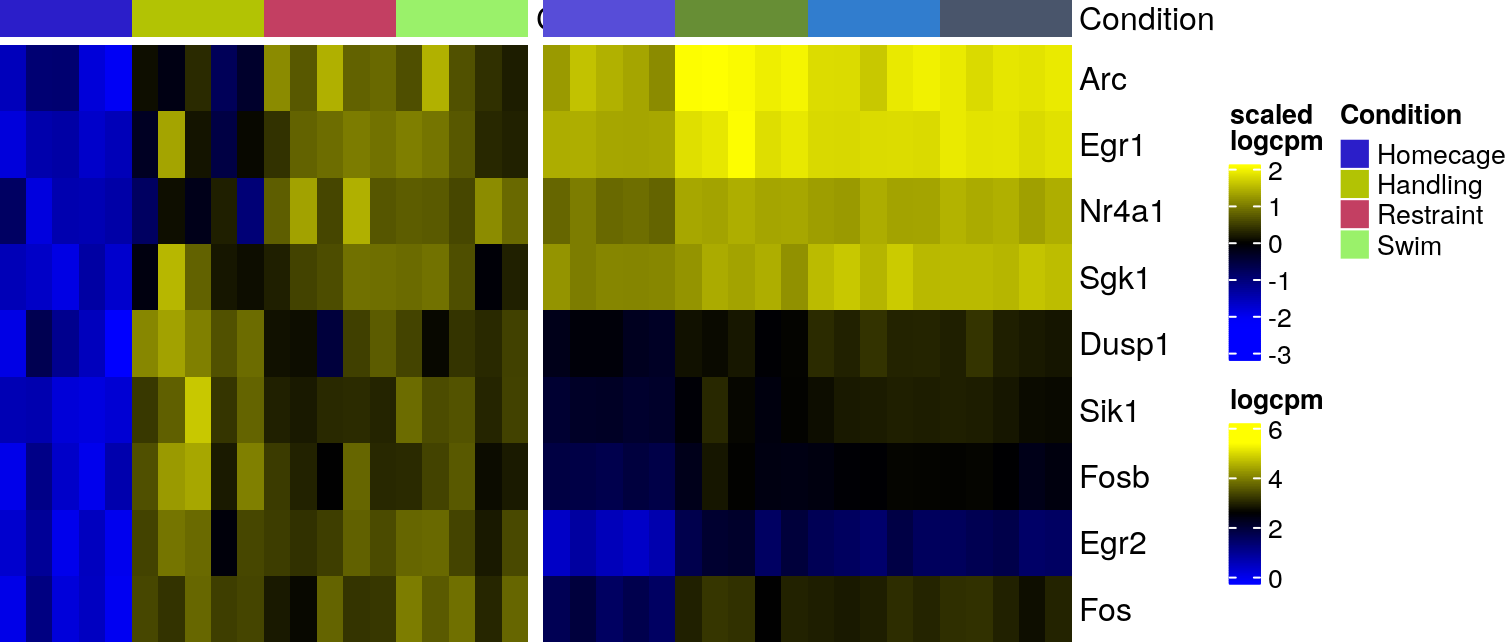However, doing so involves manual work to ensure that the labels and colors are nice and coherent, and that the rows names match. As a convenience, we provide the `crossHm` function to handle these issues. `crossHm` works with a list of `SummarizedExperiment` objects:

``````# we build another SE object:
SE2 <- SE
assays(SE2)\$logcpm <- jitter(assays(SE2)\$logcpm, factor=1000)
crossHm(list(SE1=SE, SE2=SE2), g, do.scale = TRUE)``````
``````## Using assay logcpm
## Using assay logcpm``````